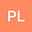•In this work, we deal with the existence and uniqueness of solutions for some classes of singular integral equations with convolution in the case of non-normal type. To obtain the conditions of Noethericity, we establish the regularity theory of solvability for the equations. By means of the theory of Fourier analysis, we transform such equations into Riemann boundary value problems. The analytic solutions and the conditions of solvability are obtained in class $\{0\}$. In particular, we discuss the asymptotic property of solutions at nodes. Therefore, our work generalizes ones in Refs.[1,2,4,5,11,12,15] and improves the theories of integral equations and the classical Riemann boundary value problems.